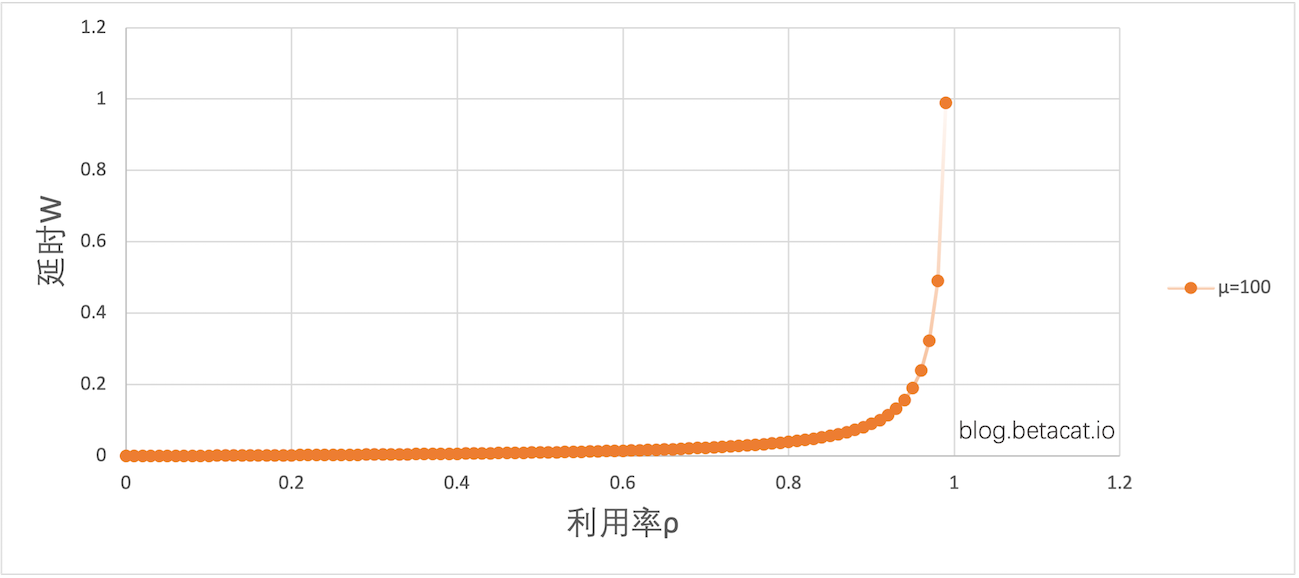## 1. 预备知识

### 1.1. Little’s Law

Little’s Law 是排队论中的一个基本定理，这里的 Little 完全是字面意思，取自于作者 John D.C. Little ，跟逻辑意义上的大小无关。它用于描述一个处于稳定状态下的排队系统中请求数、到达率以及平均逗留时间之间的关系：

$$L = λW$$

• L：系统中的平均请求数（同时包括正在接受服务的请求和等待服务的请求）。
• λ：单位时间内到达系统的请求数量，即到达率。
• W：请求在系统中的平均逗留时间（包括逗留时间和服务时间），即我们感知到的延迟。

### 1.2. M/M/1 模型

M/M/1 模型是最基本的排队模型，其中的第一个M（Markovian）表示到达率服从泊松分布（Poisson Distribution），第二个M表示服务速率也服从泊松分布，而数字1表示系统只有一个服务器。在这个模型中，平均逗留时间W可以通过以下公式计算：

$$W = \frac{λ} {μ * (μ - λ)}$$

#### 1.2.1 推导过程

M/M/1 模型的公式可以用归纳法推导出来：

 1  λ * P(X=i) = μ * P(X=j) 

 1 2 3 4  λ * P0 = μ * P1 μ * P1 = λ * P2 λ * P2 = μ * P3 ... 

 1 2 3 4 5  P1 = (λ/μ) * P0 P2 = (λ/μ)^2 * P0 P3 = (λ/μ)^3 * P0 ... Pi = (λ/μ)^i * P0 

 1  P0 + (λ/μ) * P0 + (λ/μ)^2 * P0 + ... = 1 

 1  P0 × (1 + (λ/μ) + (λ/μ)^2 + ...) = P0 × (1 / (1 - λ/μ)) = 1 

 1  P0 = 1 - λ/μ = (μ - λ) / μ 

 1  L = Σ i * P(X=i) = Σ i * (λ/μ) ^ i * P0 

 1  L = P0 * (λ/μ) * (1 + 2*(λ/μ) + 3*(λ/μ)^2 + ...) 

 1  (1 + 2*(λ/μ) + 3*(λ/μ)^2 + ...) = 1 / (1 - λ/μ)^2 

 1  L = P0 * (λ/μ) * (1 / (1 - λ/μ)^2) 

 1  L = ((μ - λ) / μ) * (λ/μ) * (1 / (1 - λ/μ)^2) 

 1  L = λ^2 / (μ * (μ - λ)) 

 1  W = L / λ = λ / (μ * (μ - λ)) 

### 1.3. 系统利用率

$$ρ = \frac{λ} {μ}$$

## 2. 串联起来

$$W = \frac{ρ} {μ * (1 - ρ)}$$

### 2.1. 感性认识0.2 0.0025 -
0.3 0.0042 68%
0.4 0.006 42%
0.5 0.010 66%
0.6 0.015 50%
0.7 0.023 53%
0.8 0.040 73%
0.9 0.090 125%
0.99 0.99 1000%

## 3. 总结

• 系统处理能力（μ）与延迟（W）成反比，提升处理能力后，也能线性的减少访问延迟。
• 吞吐与延迟成正比，所以有人认为，同一个集群不可能同时满足低延迟和高吞吐的需求，只能做取舍，具体解释如下：
• 低延迟意味着低利用率，即希望系统没有排队现象，请求来了立即就得到处理，无需等待。
• 高吞吐意味着高使用率，即希望系统队列中始终有可用请求，这样各种资源才不会有空闲的时间，系统始终处于忙碌状态。